Courses
Courses for Kids
Free study material
Free LIVE classes
More

# The Fish Tale Class 5 Notes CBSE Maths Chapter 1 (Free PDF Download)LIVE
Join Vedantu’s FREE Mastercalss

## NCERT Class 5 Chapter 1 The Fish Tale Revision Notes

The CBSE Class 5 Maths syllabus lays a foundation for advanced mathematical operations with the introduction of several basic concepts. The first chapter deals with stories related to fish. It teaches students arithmetic concepts related to time and distance, price and amount, using certain examples. Download and refer to The Fish Tale revision notes prepared by our experts to understand these concepts easily.

These notes are compiled following the CBSE Class 5 standards in simple language. Students will be able to determine the prime objective of this chapter by going through these revision notes. They will also learn to solve the questions of this chapter easily by practicing The Fish Tale Class 5 worksheets provided with the revision notes.

Last updated date: 28th Sep 2023
Total views: 144.6k
Views today: 1.44k

## Revision Notes Class 5 Mathematics Chapter 1 - The Fish Tale

### Size of the Fish

• Fishes can have very different sizes. The smallest fish size is 1cm and the largest size is approximately 18 m long.

• Largest fish in the world is the whale shark.

• Whale fish are different from other fishes. Whales breathe through their noses just like humans, not like other fishes.

• Fishes have no noses; they take in water, not air.

• Whales give birth to babies but other fishes lay eggs.

• Whale sharks look very big and dangerous but they are pretty harmless.

• In (Malayalam) Kerala fish are called “meen”.

Solved Example 1.  If the weight of one child is 20 kg, a shark is equal to 12 children like you put together will weigh about …?

Sol: Weight of one child = 20 kg.

Then weight of 12 children = 20 kg 12 = 240 kg.

The weight of 12 children is equal to one shark.

So, the weight of one shark = 240 kg.

### The Shape of the Fish

• The Shapes of fishes are combinations of the shape of triangle, square and circle shape. In fish shape the triangle is the tail of the fish, the square is the body and the circle is the eye of the fish.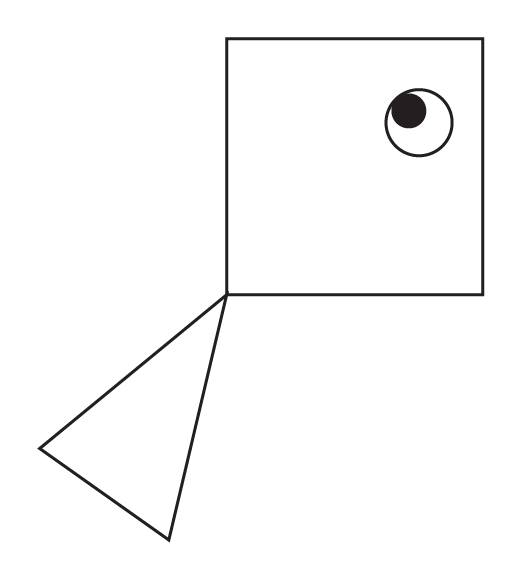Eye of the fish

• Example: Different shapes of fish.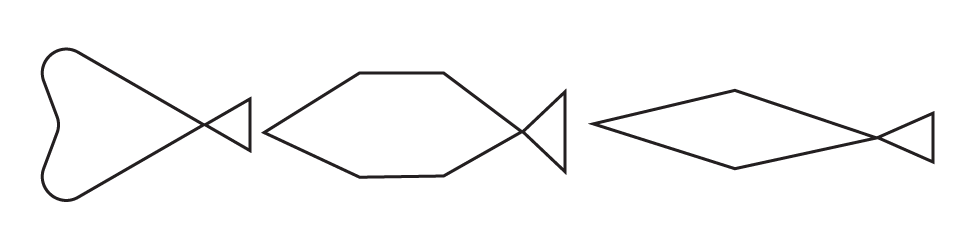Different shapes of fish

### Difference Between Whale Tail and Another Fishtail.

 Whale Other fish Whale’s tails almost look like two legs Fishtail stands flat along its body.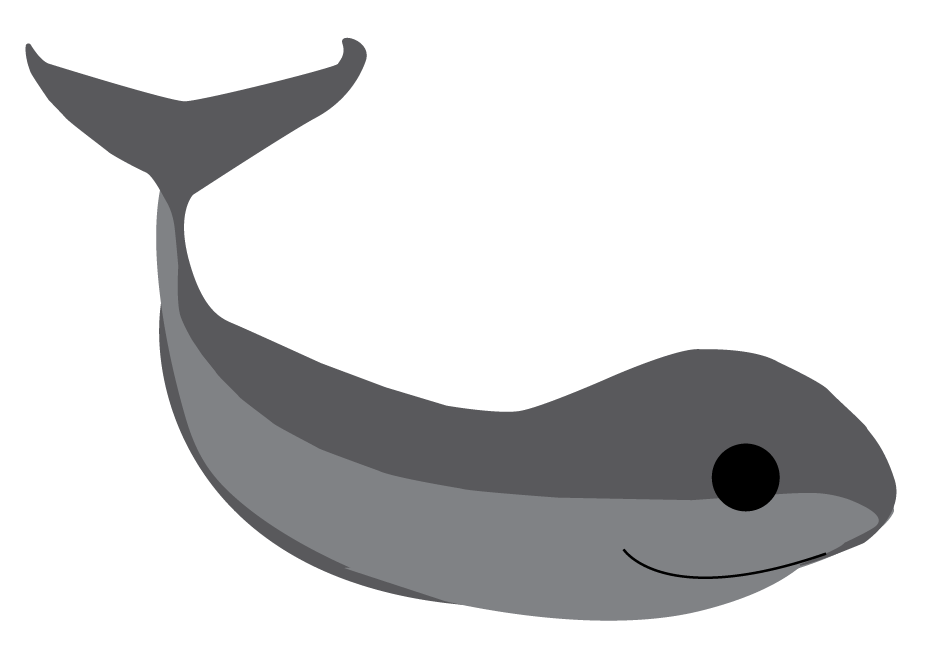### School of Fish

• When fish swim together in the river or sea in big groups called “School” of fish.

• In school they feel secure and safe.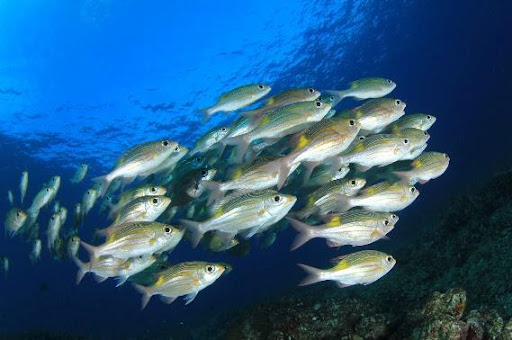School of fish

### Boat

• Fishermen use boats to go to the sea and they are going up and down with waves.

• There are different types of boats around the world. And a simple boat made from logs of wood tied together.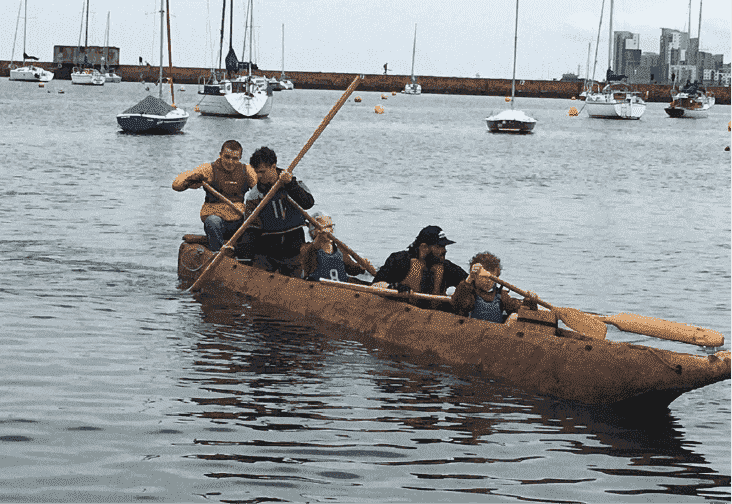Log Boat

• Fishermen use the sun for their direction sometimes they won’t get direction also.

• Boat speed is different in different types of boats. Some boats have motors and their speed is approx 20 km in one hour.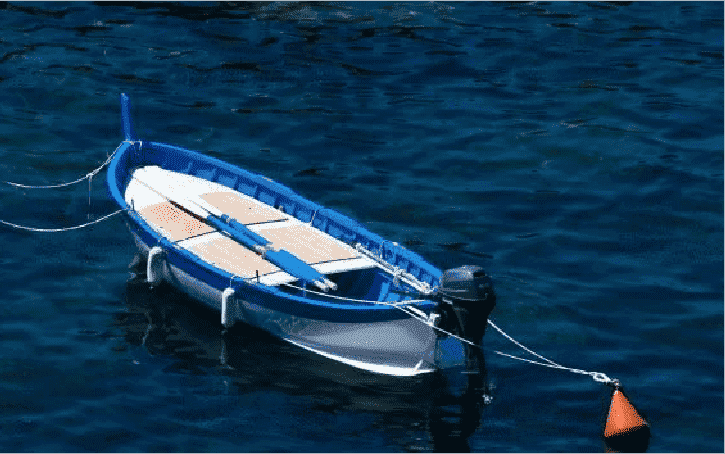Motor boat

• Fishermen use boats to catch fish by using nets.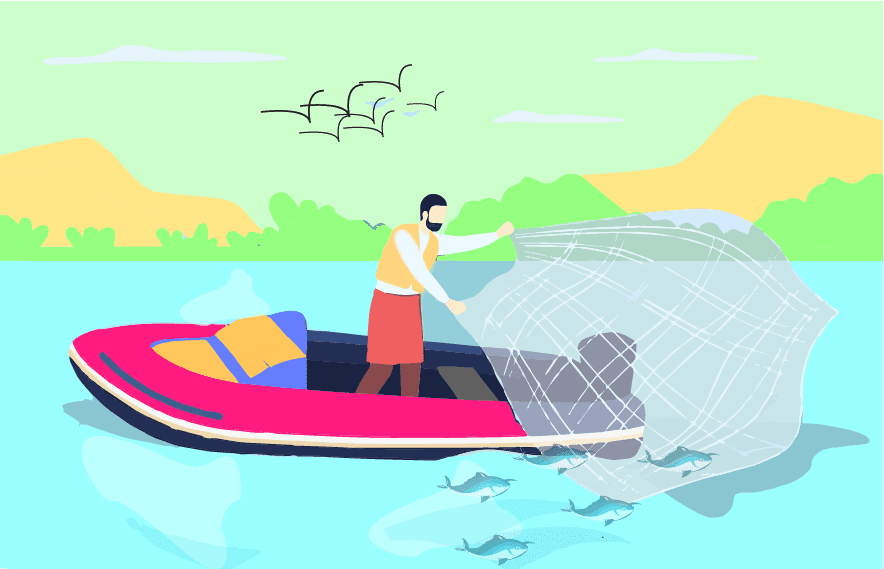Catch fish by using nets

Solved Example 2: How long it will take to go a distance of 20 km. A log boat can travel 4 km in one hour.

Ans: Speed of a log boat = 4 km per hour.

log boat travel in two hour = 4 km $\times$ 2 = 8 km.

log boat travel in three hour = 4 km $\times$ 3 = 12 km.

log boat travel in three hour = 4 km $\times$ 4 = 16 km.

Then log boat travel in Five hour = 4 km $\times$ 5 = 20 km.

Therefore log boats will take 5 hours to complete a distance of 20 km.

Solved Example 3: How long it will take to go a distance of 100 km. If a motorboat can travel 20 km in one hour.

Ans: Speed of a Motorboat = 20 km per hour.

Motor boat travel in two hour = 20 km $\times$ 2 = 40 km.

Motor boat travel in three hour = 20 km $\times$ 3 = 60 km.

Motor boat travel in three hour = 20 km $\times$ 4 = 80 km.

Then Motor boat  travels in Five hour = 20 km $\times$ 5 = 100 km.

Therefore log boats will take 5 hours to complete a distance of 100 km

### Different Types Of Boats and Their Capacity To Carry Fish and Their Speed.

 Type of Boat Capacity To Carry Fish (In Kg) Speed Logboat 20 4 km in one hour Long-tail boat 600 12 km in one hour Motorboat 800 20 km in one hour Machine boat 6000 22 km in one hour

### Estimation of Numbers

• In maths, estimation means the near value of any number and quality.

• Look at the number estimation on the number line.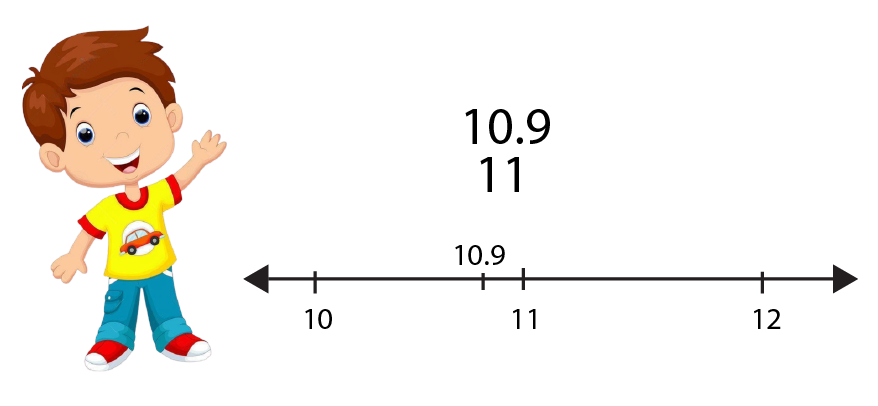Number 10.9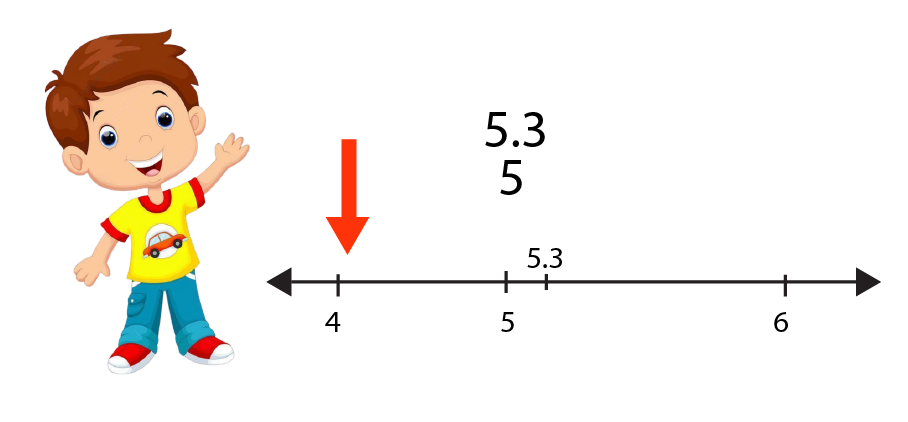Number 5.3

Example

 Exact Number Estimated Number 16.74 16.70 5.3 5.0 10.9 11.0

### Practice Question

Q1. Shivam took a loan of Rs.6000 to buy a net. He paid back Rs. 540 every month for one year. How much money did he pay back to the bank?

Ans:  Rs. 6480

Q2. Name two things that are lying to your east.

Q3. How much time will the boat take to reach 100 km, if the boat speed is 25 km in one hour?

Ans: 4 Hour.

## Importance of CBSE Class 5 Maths Chapter 1 The Fish Tale

• This chapter helps students to recall the comparison of weights they learned in the previous classes. Apart from the knowledge of marine life, students will learn how to compare their weights with that of fish.

• They will also learn to determine the information given in the textual parts and solve problems in the given exercises. The methods of extracting information from sums are explained in this chapter.

• Students will learn from The Fish Tale Class 5 notes about the concepts related to time and distance. They will also be able to determine the capacities of boats used by fishermen to catch and carry fish.

• They will also learn how to find out data from the given charts and solve the questions asked in the exercises.

## Benefits of The Fish Tale Worksheet and Revision Notes

• The subject matter experts at Vedantu have compiled the free version of The Fish Tale Class 5 PDF to explain the concepts covered in this chapter. You can refer to the revision notes to understand the objective of this chapter easily.

• Time and distance are explained by our subject experts. You can relate to the concepts and use problem-solving methods to solve the sums by picking the right information from the textual parts.

• Solving The Fish Tale Class 5 Maths PDF worksheet will also help you sharpen your skills. Refer to the NCERT Solutions provided on Vedantu to understand the accurate methods to solve and practise the sums given in this chapter.

## Understanding the Concepts of the Fish Tale with Notes

Download the Class 5 Maths Chapter 1 The Fish Tale worksheet and revision notes for free from Vedantu. Refer to these revision notes to revise the concepts effectively before the exam. Going through these notes and practising the worksheets will help you to sharpen your problem-solving skills. Thus you can follow the solutions given here by our subject experts to solve the sums correctly and score well in the exams.

## FAQs on The Fish Tale Class 5 Notes CBSE Maths Chapter 1 (Free PDF Download)

1. How can I practice to draw a fish?

Follow the instructions given in NCERT Maths Class 5 Chapter 1, and proceed to use the steps given in the revision notes to practice and learn how to accurately draw a fish.

2. How can I solve problems related to the fishermen in their boats for Class 5 Maths Chapter The Fish Tale?

Focus on how we have drawn information from the problems given in the NCERT Solutions for Class 5 Maths Chapter 1. Download this PDF and refer to the solutions to find out where you need to work more and check the steps involved in the solution for each type of question covered in this chapter. Practice solving the worksheets provided in the revision notes. This is how you can solve the problems related to the fishermen in Class 5 The Fish Tale.

3. What is the best way to solve fish market problems?

Check the price of the fish sold in a market for a given problem sum. Find out what you have to answer and utilize the methods of multiplication and division to find the answers accurately.